Courses

# Test: Method of Analysis

## 10 Questions MCQ Test Analog Electronics | Test: Method of Analysis

Description
This mock test of Test: Method of Analysis for Electrical Engineering (EE) helps you for every Electrical Engineering (EE) entrance exam. This contains 10 Multiple Choice Questions for Electrical Engineering (EE) Test: Method of Analysis (mcq) to study with solutions a complete question bank. The solved questions answers in this Test: Method of Analysis quiz give you a good mix of easy questions and tough questions. Electrical Engineering (EE) students definitely take this Test: Method of Analysis exercise for a better result in the exam. You can find other Test: Method of Analysis extra questions, long questions & short questions for Electrical Engineering (EE) on EduRev as well by searching above.
QUESTION: 1

### V1 = ?​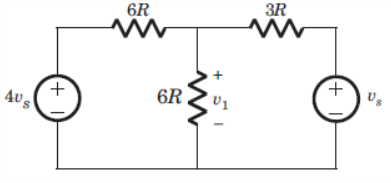Solution:

Apply nodal analysis, V1 = Vs(4/6 + 1/3)/(1/6 + 1/3 + 1/6) = 1.5 Vs.

QUESTION: 2

### Va = ?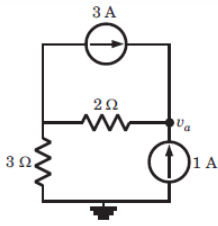Solution:

Va = 2(3+1) + 3 = 11 V.

QUESTION: 3

### V1 = ?​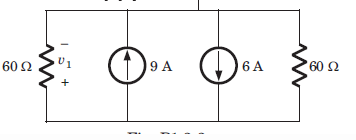Solution:

-V1/60 – V1/60 + 6 = 9 or V1 = -90 V.

QUESTION: 4

Va = ?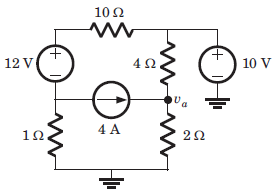Solution:

(Va – 10)/4 + Va/2 = 4 or Va = 8.67 V.

QUESTION: 5

If there are 8 nodes in network, we can get ____ number of equations in the nodal analysis.

Solution:

Number of equations=N-1= 7. So as there are 8 nodes in network, we can get 7 number of equations in the nodal analysis.

QUESTION: 6

i1 = ?​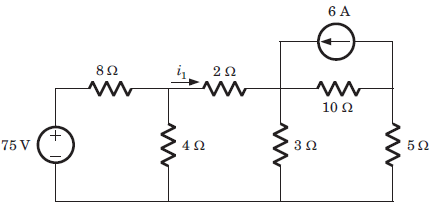Solution:

i1 = 10/36+64 + 0.5 => i1 = 0.6 A.

QUESTION: 7

Ib = ?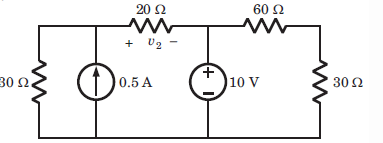Solution:
QUESTION: 8

i1 = ?​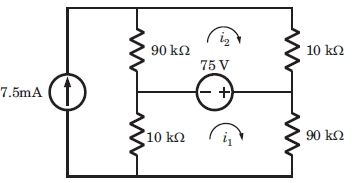Solution:

75 = 90k (i1 – 7.5m) => 150 = 100ki1 => i = 1.5 mA.

QUESTION: 9

i1 = ?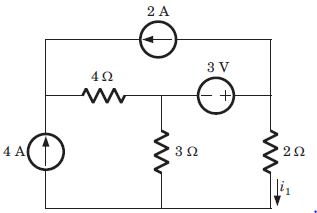Solution:

3 – 5i1 – 12 => i1 = 3A.

QUESTION: 10

i1 = ?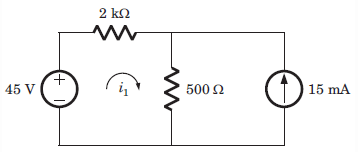Solution:

45 = 2k*i1 + 500(i1 + 15*10-3) => i1 = 15 mA.